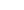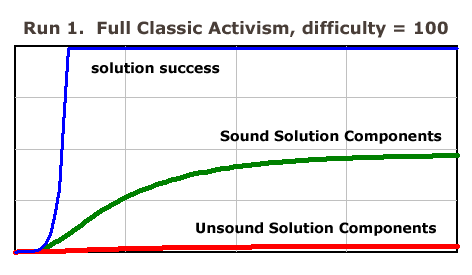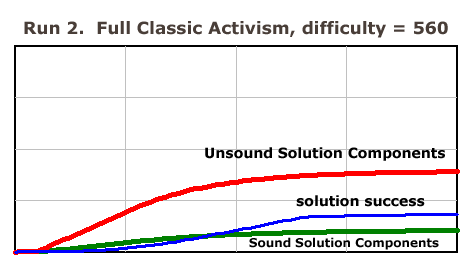# The Memetic Evolution of Solutions to Difficult Problems

This 15 page section PDF from the Analytical Activism book is titled The Memetic Evolution of Solutions to Difficult Problems. It presents a simulation model that demonstrates how solution memes evolve.

While solutions to easy problems follow predictably from the application of known principles and procedures, solutions to difficult problems take an entirely different route. They evolve. This section presents a simulation model that uses memes, the evolutionary algorithm, and the Scientific Method to explain how solutions to difficult problems evolve and how the process can be improved.

The key point is that today most sustainability solution evolution is haphazard, unpredictable, costly, slow, and frustratingly ineffective. And it's been that way for decades. But there is a better way. It involves turning the process of solution evolution into one that is orderly, predictable, cost effective, fast, and highly effective. As the model shows, this can be done.

The heart of the model is the use of the three steps of the evolutionary algorithm: (1) mutation, (2) selection, and (3) replication. Here's how the model handles these steps:

Step 1. Mutation - A solution meme starts its life cycle as a Hypothesis to Test. All hypotheses are memetic mutations of what came before them, so this is the first step of the evolutionary algorithm.

Step 2. Selection - Next experiments are run to test the backlog of hypotheses memes. The memes flow into Experiments Completed, where they are then reviewed. This is where selection, also known as survival of the fittest, occurs. A hypotheses meme is either accepted (it lives), rejected (it dies), or modified. If modified it then goes back to step one, because it is a new hypotheses. Memes that survive the selection step flow into Hypotheses Accepted.

At this point the model takes a novel direction. It recognizes that to err is human, so all accepted hypotheses contain a mixture of sound (true) and unsound (false) memes. Experimentation can only say that a hypothesis meme has a high probability of being true. It can never say with absolute certainty that anything is true. This is a crucial distinction, because the ratio of sound memes to total memes in a solution determines the soundness of the solution and whether is it probably going to work or not.

How good an organization is at solving difficult problems depends on one main thing: how efficient its problem solving process is. If its process is incapable of producing a solution with a high percentage of sound memes, then it will be unable to reliably solve difficult problems, even if a large amount of effort is expended. Regrettably, it appears this is where most environmental organizations are today.

The model divides accepted hypotheses memes into two groups: Unsound Selections and Sound Selections. Each are then available for the next step, which is:

Step 3. Replication - In this step the unsound and sound memes are transmitted to the user community as solution components, which are used to solve the problem. Solution success occurs if there is a high percentage of sound memes in the solution. The more difficult the problem, the higher this percentage must be and the larger the number of sound memes needed to solve the problem.

## The Simulation Runs

Using 11 simulation runs, the model explores how the process of memetic solution evolution works and how it can be improved, so that environmentalists can begin to solve the very difficult problems they face today, particularly the toughest and most urgent one of them all: climate change.

Let's briefly look at the first two simulation runs, so you can get a feel for how the model works. The first run is shown below. The vertical axis is percent solution success and number of solution components, and the horizontal axis is time.A simulation run is a scenario of how the system behaves under certain conditions. Run 1 assumes the process being used to solve the problem is Classic Activism and that the problem being worked on has a difficulty of 100 on a scale of 0 to 1,000. It is an easy problem, so Classic Activism works. Classic activists are able to produce enough Sound Solution Components to solve the problem. (A component is a meme.) The overall solution contains such a small percentage of Unsound Solution Components that they do not affect Solution Success, which is very high. But the next run shows a different story.The conditions for run 2 are the same as for run 1 with one change: problem difficulty has increased from 100 to 560. The classic activists are working on a medium difficulty problem. The graph shows how Classic Activism is not a good enough process to solve medium difficulty problems, because it produces about three times as many Unsound Solution Components as Sound Solution Components.

Examples of medium difficulty problems are national pollution and regional natural resource depletion. Historically, medium size problems tend to be solved poorly or not at all until after several tries. These problems are well past the ability of Classic Activism to solve reliably, so the model reflects that.

The dominant paradigm today in the environmental movement is Classic Activism. The rest of the simulation runs explore how this can be changed one element at a time to Analytical Activism, which is fully capable of solving problems of high difficulty.

## The Simulation Model

The main portion of the model is shown below. To read it, first study the seven stocks (the boxes). These contains pools of particular types of memes. The memes flow through the system in the pipes connecting the stocks. Memes are created or die in the little clouds. How the memes flow is controlled by the many variables in the model, each of which represents a particular rule of behavior in the real world. This is a stock and flow model, the same kind used so successfully in the Limits to Growth model of 1972 and in many other projects.

The model is simpler than it looks, and is fully explained in the PDF file download at the top of this page. The actual model used in the chapter is available here.